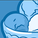# QlikView App Dev

Discussion Board for collaboration related to QlikView App Development.

Announcements
Join “Do More With Qlik” with Mike Tarallo to get a first-hand look at the New Authoring Experience, June 29th: REGISTER HERE
cancel
Showing results for
Did you mean:Creator II

## Set different color depending on the variable's value

Hello guys,

I created a variable called vAirComp1, with the following expression in order to collect the first item selected on the field called NCIA.

``=SubField(GetFieldSelections(NCIA, ' - ', 5),' - ',1)``

And once the user can select as many NCIAs as possible, I'm using the following background color expression in order to color my chart line based on the user's selection (vAirComp1 value).

``````if(vAirComp1='AZU',RGB(14,43,88),
if(vAirComp1='GLO',RGB(255,112,32),
if(vAirComp1='PTB',rgb(255,213,3),
if(vAirComp1='ARG',rgb(0,173,239),
if(vAirComp1='SKU',rgb(89,35,127),
if(vAirComp1='VVC',rgb(255,219,0),
if(vAirComp1='EFY',rgb(254,118,17),
if(vAirComp1='BOV',rgb(13,42,98),
if(vAirComp1='BWA',rgb(175,38,120),
if(vAirComp1='RPB',rgb(103,51,205),
if(vAirComp1='NSE',rgb(211,52,53),
if(vAirComp1='SRU',rgb(74,90,97),
if(vAirComp1='FBZ',rgb(254,190,16),
if(vAirComp1='THT',rgb(45,204,211),
if(vAirComp1='VTA',rgb(223,0,43),
if(vAirComp1='ECO',rgb(0,173,238),
if(vAirComp1='ACL',rgb(108,18,64),
if(vAirComp1='VCV',rgb(239,126,42),
if(vAirComp1='DAP',rgb(45,45,46),
if(vAirComp1='ROR',rgb(253,230,0),
if(vAirComp1='RYL',rgb(11,70,150),
if(vAirComp1='SRC',rgb(38,73,110),
if(vAirComp1='TGY',rgb(0,35,93),
if(vAirComp1='SID',rgb(47,57,117),
if(vAirComp1='MWM',rgb(147,200,70),
if(vAirComp1='JAT',rgb(158,32,45),
if(vAirComp1='WAY',rgb(103,51,205),
if(vAirComp1='AZN' or vAirComp1='AZP',rgb(0,166,81),
if(vAirComp1='AVA' or vAirComp1='TPA',rgb(2219,19,25),
if(vAirComp1='LAN' or vAirComp1='LCO',rgb(230,51,88),
))))))))))))))))))))))))))))))``````

But as you can see, there are lots of IFs statements...

Is there any better way of doing this?
I'm pretty sure I'm not using the smartest solution... 😁

Any ideas?

Thanks

3 RepliesMVP

You can try with Pick(Match()) like below

Pick(Match(vAirComp1, 'AZU', 'GLO'), RGB(14,43,88),RGB(255,112,32))

Or, you can bring color field in the script & use it in front end.

Thanks & Regards,
Mayil Vahanan RCreator II
Author

Hello Mr. @MayilVahanan, how are you?

I don't think doing something like this "Pick(Match(vAirComp1, 'AZU', 'GLO'), RGB(14,43,88),RGB(255,112,32))" will improve performance because I've lots of different airlines, about 40 different vAirComp1, so 40 different rgb colors.

I'm already using a script where I created an inline load statement, and defined a key field and its colors... something like this:

``````LOAD * INLINE [
NCIA,AIRN,AIRC,RRRR,GGGG,BBBB
AJB,American Jet,Argentina,120,45,145
...
NAA,Norwegian Air Argentina,Argentina,216,25,57
AZN,Amaszonas,Bolivia,0,166,81
...
VNE,Venezolana,Venezuela,11,175,42
];``````

So, everytime the user selects an specific NCIA, I'm able to college its associate color.

My variable called vAirComp1 is related somehow to NCIA, and it's defined as:

``=SubField(GetFieldSelections(NCIA, ' - ', 5),' - ',1)``

My intention is to set the colors of vAirComp1 (depending on its value) as already defined on my inline load statement... but I have no idea how to do it.

What do you think about it?
Is it possible to work with colors and variables on load script?

ThanksMVP